Q-Chem 5.0 User’s Manual

# 5.3 Møller-Plesset Perturbation Theory

## 5.3.1 Introduction

Møller-Plesset Perturbation Theory  is a widely used method for approximating the correlation energy of molecules. In particular, second-order Møller-Plesset perturbation theory (MP2) is one of the simplest and most useful levels of theory beyond the Hartree-Fock approximation. Conventional and local MP2 methods available in Q-Chem are discussed in detail in Sections 5.4 and 5.5 respectively. The MP3 method is still occasionally used, while MP4 calculations are quite commonly employed as part of the G2 and G3 thermochemical methods [288, 289]. In the remainder of this section, the theoretical basis of Møller-Plesset theory is reviewed.

## 5.3.2 Theoretical Background

The Hartree-Fock wave function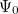and energy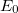are approximate  solutions (eigenfunction and eigenvalue) to the exact Hamiltonian eigenvalue problem or Schrödinger’s electronic wave equation, Eq. (4.5). The HF wave function and energy are, however, exact solutions for the Hartree-Fock Hamiltonian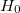eigenvalue problem. If we assume that the Hartree-Fock wave functionand energylie near the exact wave function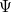and energy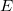, we can now write the exact Hamiltonian operator as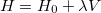(5.1)

where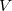is the small perturbation and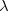is a dimensionless parameter. Expanding the exact wave function and energy in terms of the HF wave function and energy yields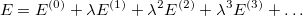(5.2)

and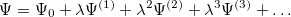(5.3)

Substituting these expansions into the Schrödinger equation and collecting terms according to powers ofyields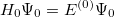(5.4)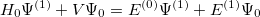(5.5)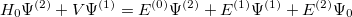(5.6)

and so forth. Multiplying each of the above equations byand integrating over all space yields the following expression for theth-order (MP) energy: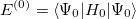(5.7)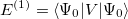(5.8)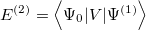(5.9)

Thus, the Hartree-Fock energy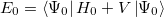(5.10)

is simply the sum of the zeroth- and first- order energies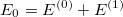(5.11)

The correlation energy can then be written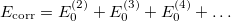(5.12)

of which the first term is the MP2 energy.

It can be shown that the MP2 energy can be written (in terms of spin-orbitals) as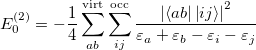(5.13)

where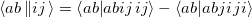(5.14)

and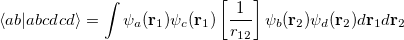(5.15)

which can be written in terms of the two-electron repulsion integrals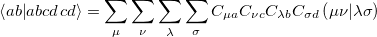(5.16)

Expressions for higher order terms follow similarly, although with much greater algebraic and computational complexity. MP3 and particularly MP4 (the third and fourth order contributions to the correlation energy) are both occasionally used, although they are increasingly supplanted by the coupled-cluster methods described in the following sections. The disk and memory requirements for MP3 are similar to the self-consistent pair correlation methods discussed in Section 5.8 while the computational cost of MP4 is similar to the (T) corrections discussed in Section 5.9.